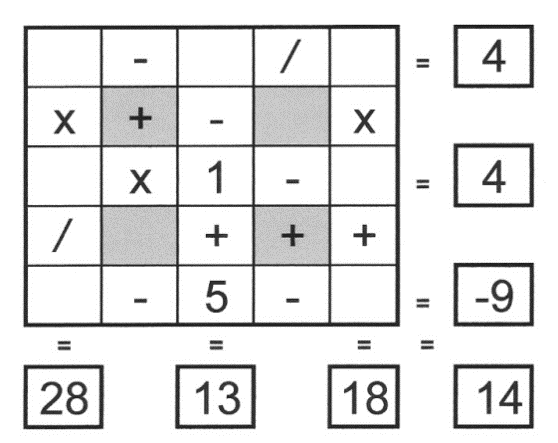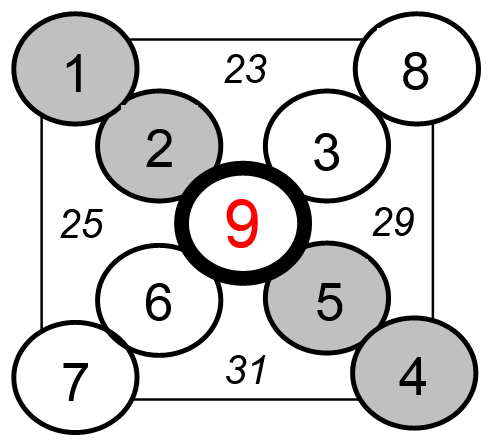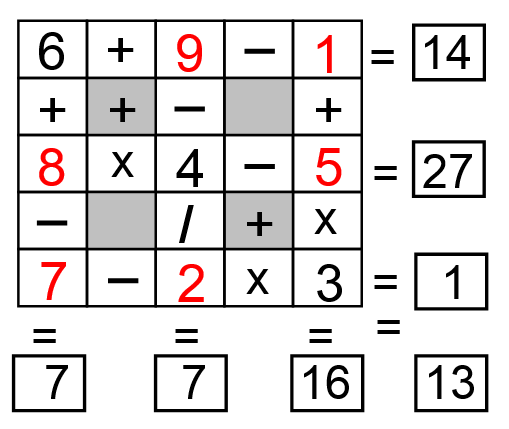Select Page

Each month, a new set of puzzles will be posted.  Come back next month for the solutions and a new set of puzzles, or subscribe to have them sent directly to you.

## MIND-Xpander maths problem

If N is a whole number and the following limits apply:  2N > 30 and 5N < 100, what values for N are possible?

## Petite CIRCLE-Sums puzzles

The number within the 4 sectors of the outer circle is equal to the sum of the three numbers in its sector. The numbers in the individual circles can only be 1 to 9 and each number can be used only once. One number has been provided to get you started. Find the remaining 4 numbers. Note: There may be more than one solution.## EQUATE+2 puzzle

Each row, column & diagonal is an equation and you use the numbers 1 to 9 to complete the equations. Each number can be used only once. Two numbers have been provided to get you started. Find the remaining seven numbers that satisfy all the resulting equations. Note – multiplication (x) & division (/) are performed before addition (+) and subtraction (-).## Feedback

There are more than one way of doing these puzzles and may well be more than one answer.  Please let me and others know what alternatives you find by commenting below.  We also welcome general comments on the subject and any feedback you'd like to give.

If you have a question that needs a response from me or you would like to contact me privately, please use the contact form.

## Get more puzzles!

If you've enjoyed doing the puzzles, consider ordering the book; 150+ of the best puzzles in a handy pocket sized format. Click here for full details.

## MIND-Xpander Logic Problem:

In basic algebra, a rational expression is nothing more than a fraction in which the numerator and/or the denominator are polynomials. Reduce the following rational expression to its lowest terms.65 – 64  =  (6 x 64) – (1 x 64)  =  (6 – 1) (64)  =  5 x 64

Now, dividing by 5 will give the answer of 64

## X-Sums Puzzles

The 4 numbers, in italics, outside the 9 circles represent the sum of the numbers in the 5 surrounding circles. The numbers in the circles can only be 1 to 9 and each number can be used only once. Four numbers have been provided to get you started. Find the number (?) that should be in the centre of the X.## EQUATE+3 Puzzle

Each row, column & diagonal is an equation and you use the numbers 1 to 9 to complete the equations. Each number can be used only once. ‘Three’ numbers have been provided to get you started. Find the remaining six numbers that satisfies all the resulting equations. Note – multiplication (x) & division (/) are performed before addition (+) and subtraction (-).